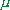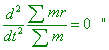V.6 No 1 67 The problem of physical time in today physics6.3. Mach’s absolutisation of relative orbital motion Having sorted out Einsteinian treatment of Mach’s principle of relativity, consider this issue in the original statement of Mach which he stated in his “Mechanics”. In brief, he stated it so: “I think existent only relative motion… and I cannot assume here any difference between the orbital and forward motion. If the body revolved relative to the sky of resting stars, the centrifugal forces arise, and if it revolved relative to another body, not relatively the sky of resting stars, centrifugal forces are absent. I am not against to call the first motion absolute, only if we do not forget that it means nothing else than revolving relative to the sky of resting stars. Can we, holding the Newtonian vessel with water being at rest, revolve the sky with stars relative to it and prove the absence of centrifugal forces in this case?” [61, p. 60]. So Mach raised the issue of relative orbiting motion which is well deeper than relativists interpreted it. So deep that it made many adherents of classical mechanics even to deny partially the Newtonian principles in some models containing the orbiting: “the acceleration of material point relative to a non-inertial reference frame arises under affection of the force F from a definite body (or bodies), as well as resulting from the accelerated motion of the frame S'  relative to the inertial frame S. We can treat the acceleration of material point relative to the non-inertial reference frame as the result of inertial forces. We cannot point for these forces the source as a definite body affecting this material point. So this force of inertia has not a related counteracting force, in other words, the forces of inertia, as opposite to the forces of interaction, do not obey the third Newton law” [62, p. 166]. With it all, if we approach the issue of relative orbiting raised by Mach with all thoroughness which the classical formalism allows, we will reveal that Newton was right stating so: “The effects which distinguish absolute from relative motion are the forces of receding from the axis of circular motion. For there are no such forces in a circular motion purely relative, but in a true and absolute circular motion, they are greater or less, according to the quantity of the motion” [2, p. 34]. To prove Newton’s conclusion, let us understand more precisely, what namely he meant separating the orbiting into the absolute and relative. Giving an example of the vessel with water, Newton explained it as follows: “If a vessel, hung by a long cord, is so often turned about that the cord is strongly twisted, then filled with water, and held at rest together with the water; after, by the sudden action of another force, it is whirled about the contrary way, and while the cord is untwisting itself, the vessel continues, for some time in this motion; the surface of the water will at first be plain, as before the vessel began to move ” [2, p. 34]. In other words, while the walls of vessel involve the water into orbiting due to the liquid friction, and so all its parts remain resting relative to the inertial frame in which we are experimenting, the motion of pail with respect to water is relative, as only pail moves, not the water in it. And the water not necessarily has to be just in the vessel. The same relative orbiting would take place if the water was in the vessel that stands near the orbiting vessel, or would be put into the vessel that is put into another vessel that orbits and has no constraint with this first. Naturally, the forces of inertia do not arise in the liquid with it, as the liquid rests, but in the orbiting vessel these forces exist, and if the orbiting is too fast, the vessel can destroy, which would evidence the presence of these forces of inertia affecting the vessel, not the liquid in it. Here we also find the disproof of Mach’s premise that the inertia forces can be substituted by the attraction of stars which he formulated in the following way: “instead to interrelate the moving body K to the space (to some coordinate system), let us consider its immediate relation to the bodies of the world through which we only can determine the coordinate system … Instead saying, the distance and speed of mass in space remain constant, we can use the expression that the average acceleration of the massrelative to the masses m , m' , m'' , ...  located at distances r , r' , r''  , ...  is zero, or(6.17)